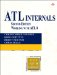Function Templates

 Classes are not the only thing that can be parameterized; functions can be, too. The canonical example is the min function: inline long min(long a, long b) { return (a < b ? a : b); } inline float min(float a, float b) { return (a < b ? a : b); } Because the code is the same for both overloaded min implementations, there's no reason not to make it into a template: template inline T min(T a, T b) { return (a < b ? a : b); } When the template is instantiated, the compiler generates the code on demand based on the use: void main() { // produces: long min(long, long) long a = 1, b = 2, c = min(a, b); // produces: float min(float, float) float x = 1.1, y = 2.2, z = min(x, y); // produces: char *min(char *, char *) char *r = "hello", *s = "world", *t = min(r, s); } Notice that the compiler can figure out how to insatiate the min template implementation without any fancy angle brackets. However, also notice that sometimes, just as with class templates, function templates don't expand the way we'd like. For example, the min that takes two char* compares two pointer values, not the contents of the strings. A function template can be specialized by merely providing an implementation that takes the types involved: inline char* min(const char* a, const char* b) { return (strcmp(a, b) < 0 ? a : b); } In this case, because a version of the function that takes two character pointers already exists, the compiler binds to that one instead of generating another implementation based on the min function template.ATL Internals: Working with ATL 8 (2nd Edition)
ISBN: 0321159624
EAN: 2147483647
Year: 2004
Pages: 172

Similar book on Amazon